Professor Murray

Computations of Inverse Trigonometric Functions

Slide Duration:

Section 1: Trigonometric Functions
Angles

39m 5s

Intro
0:00
Degrees
0:22
Circle is 360 Degrees
0:48
Splitting a Circle
1:13
2:08
2:31
2:52
Half-Circle and Right Angle
4:00
6:24
6:52
Coterminal, Complementary, Supplementary Angles
7:23
Coterminal Angles
7:30
Complementary Angles
9:40
Supplementary Angles
10:08
Example 1: Dividing a Circle
10:38
Example 2: Converting Between Degrees and Radians
11:56
Example 3: Quadrants and Coterminal Angles
14:18
Extra Example 1: Common Angle Conversions
-1
Extra Example 2: Quadrants and Coterminal Angles
-2
Sine and Cosine Functions

43m 16s

Intro
0:00
Sine and Cosine
0:15
Unit Circle
0:22
Coordinates on Unit Circle
1:03
Right Triangles
1:52
2:25
Master Right Triangle Formula: SOHCAHTOA
2:48
Odd Functions, Even Functions
4:40
Example: Odd Function
4:56
Example: Even Function
7:30
Example 1: Sine and Cosine
10:27
Example 2: Graphing Sine and Cosine Functions
14:39
Example 3: Right Triangle
21:40
Example 4: Odd, Even, or Neither
26:01
Extra Example 1: Right Triangle
-1
Extra Example 2: Graphing Sine and Cosine Functions
-2
Sine and Cosine Values of Special Angles

33m 5s

Intro
0:00
45-45-90 Triangle and 30-60-90 Triangle
0:08
45-45-90 Triangle
0:21
30-60-90 Triangle
2:06
Mnemonic: All Students Take Calculus (ASTC)
5:21
Using the Unit Circle
5:59
New Angles
6:21
9:43
Mnemonic: All Students Take Calculus
10:13
13:11
16:48
Example 3: All Angles and Quadrants
20:21
Extra Example 1: Convert, Quadrant, Sine/Cosine
-1
Extra Example 2: All Angles and Quadrants
-2
Modified Sine Waves: Asin(Bx+C)+D and Acos(Bx+C)+D

52m 3s

Intro
0:00
Amplitude and Period of a Sine Wave
0:38
Sine Wave Graph
0:58
Amplitude: Distance from Middle to Peak
1:18
Peak: Distance from Peak to Peak
2:41
Phase Shift and Vertical Shift
4:13
Phase Shift: Distance Shifted Horizontally
4:16
Vertical Shift: Distance Shifted Vertically
6:48
Example 1: Amplitude/Period/Phase and Vertical Shift
8:04
Example 2: Amplitude/Period/Phase and Vertical Shift
17:39
Example 3: Find Sine Wave Given Attributes
25:23
Extra Example 1: Amplitude/Period/Phase and Vertical Shift
-1
Extra Example 2: Find Cosine Wave Given Attributes
-2
Tangent and Cotangent Functions

36m 4s

Intro
0:00
Tangent and Cotangent Definitions
0:21
Tangent Definition
0:25
Cotangent Definition
0:47
Master Formula: SOHCAHTOA
1:01
Mnemonic
1:16
Tangent and Cotangent Values
2:29
Remember Common Values of Sine and Cosine
2:46
90 Degrees Undefined
4:36
Slope and Menmonic: ASTC
5:47
Uses of Tangent
5:54
Example: Tangent of Angle is Slope
6:09
7:49
Example 1: Graph Tangent and Cotangent Functions
10:42
Example 2: Tangent and Cotangent of Angles
16:09
Example 3: Odd, Even, or Neither
18:56
Extra Example 1: Tangent and Cotangent of Angles
-1
Extra Example 2: Tangent and Cotangent of Angles
-2
Secant and Cosecant Functions

27m 18s

Intro
0:00
Secant and Cosecant Definitions
0:17
Secant Definition
0:18
Cosecant Definition
0:33
Example 1: Graph Secant Function
0:48
Example 2: Values of Secant and Cosecant
6:49
Example 3: Odd, Even, or Neither
12:49
Extra Example 1: Graph of Cosecant Function
-1
Extra Example 2: Values of Secant and Cosecant
-2
Inverse Trigonometric Functions

32m 58s

Intro
0:00
Arcsine Function
0:24
Restrictions between -1 and 1
0:43
Arcsine Notation
1:26
Arccosine Function
3:07
Restrictions between -1 and 1
3:36
Cosine Notation
3:53
Arctangent Function
4:30
Between -Pi/2 and Pi/2
4:44
Tangent Notation
5:02
Example 1: Domain/Range/Graph of Arcsine
5:45
Example 2: Arcsin/Arccos/Arctan Values
10:46
Example 3: Domain/Range/Graph of Arctangent
17:14
Extra Example 1: Domain/Range/Graph of Arccosine
-1
Extra Example 2: Arcsin/Arccos/Arctan Values
-2
Computations of Inverse Trigonometric Functions

31m 8s

Intro
0:00
Inverse Trigonometric Function Domains and Ranges
0:31
Arcsine
0:41
Arccosine
1:14
Arctangent
1:41
Example 1: Arcsines of Common Values
2:44
Example 2: Odd, Even, or Neither
5:57
Example 3: Arccosines of Common Values
12:24
Extra Example 1: Arctangents of Common Values
-1
Extra Example 2: Arcsin/Arccos/Arctan Values
-2
Section 2: Trigonometric Identities
Pythagorean Identity

19m 11s

Intro
0:00
Pythagorean Identity
0:17
Pythagorean Triangle
0:27
Pythagorean Identity
0:45
Example 1: Use Pythagorean Theorem to Prove Pythagorean Identity
1:14
Example 2: Find Angle Given Cosine and Quadrant
4:18
Example 3: Verify Trigonometric Identity
8:00
Extra Example 1: Use Pythagorean Identity to Prove Pythagorean Theorem
-1
Extra Example 2: Find Angle Given Cosine and Quadrant
-2
Identity Tan(squared)x+1=Sec(squared)x

23m 16s

Intro
0:00
Main Formulas
0:19
Companion to Pythagorean Identity
0:27
For Cotangents and Cosecants
0:52
How to Remember
0:58
Example 1: Prove the Identity
1:40
Example 2: Given Tan Find Sec
3:42
Example 3: Prove the Identity
7:45
Extra Example 1: Prove the Identity
-1
Extra Example 2: Given Sec Find Tan
-2

52m 52s

Intro
0:00
0:09
How to Remember
0:48
Cofunction Identities
1:31
How to Remember Graphically
1:44
Where to Use Cofunction Identities
2:52
Example 1: Derive the Formula for cos(A-B)
3:08
Example 2: Use Addition and Subtraction Formulas
16:03
Example 3: Use Addition and Subtraction Formulas to Prove Identity
25:11
Extra Example 1: Use cos(A-B) and Cofunction Identities
-1
Extra Example 2: Convert to Radians and use Formulas
-2
Double Angle Formulas

29m 5s

Intro
0:00
Main Formula
0:07
How to Remember from Addition Formula
0:18
Two Other Forms
1:35
Example 1: Find Sine and Cosine of Angle using Double Angle
3:16
Example 2: Prove Trigonometric Identity using Double Angle
9:37
Example 3: Use Addition and Subtraction Formulas
12:38
Extra Example 1: Find Sine and Cosine of Angle using Double Angle
-1
Extra Example 2: Prove Trigonometric Identity using Double Angle
-2
Half-Angle Formulas

43m 55s

Intro
0:00
Main Formulas
0:09
Confusing Part
0:34
Example 1: Find Sine and Cosine of Angle using Half-Angle
0:54
Example 2: Prove Trigonometric Identity using Half-Angle
11:51
Example 3: Prove the Half-Angle Formula for Tangents
18:39
Extra Example 1: Find Sine and Cosine of Angle using Half-Angle
-1
Extra Example 2: Prove Trigonometric Identity using Half-Angle
-2
Section 3: Applications of Trigonometry
Trigonometry in Right Angles

25m 43s

Intro
0:00
Master Formula for Right Angles
0:11
SOHCAHTOA
0:15
Only for Right Triangles
1:26
Example 1: Find All Angles in a Triangle
2:19
Example 2: Find Lengths of All Sides of Triangle
7:39
Example 3: Find All Angles in a Triangle
11:00
Extra Example 1: Find All Angles in a Triangle
-1
Extra Example 2: Find Lengths of All Sides of Triangle
-2
Law of Sines

56m 40s

Intro
0:00
Law of Sines Formula
0:18
SOHCAHTOA
0:27
Any Triangle
0:59
Graphical Representation
1:25
Solving Triangle Completely
2:37
When to Use Law of Sines
2:55
ASA, SAA, SSA, AAA
2:59
SAS, SSS for Law of Cosines
7:11
Example 1: How Many Triangles Satisfy Conditions, Solve Completely
8:44
Example 2: How Many Triangles Satisfy Conditions, Solve Completely
15:30
Example 3: How Many Triangles Satisfy Conditions, Solve Completely
28:32
Extra Example 1: How Many Triangles Satisfy Conditions, Solve Completely
-1
Extra Example 2: How Many Triangles Satisfy Conditions, Solve Completely
-2
Law of Cosines

49m 5s

Intro
0:00
Law of Cosines Formula
0:23
Graphical Representation
0:34
Relates Sides to Angles
1:00
Any Triangle
1:20
Generalization of Pythagorean Theorem
1:32
When to Use Law of Cosines
2:26
SAS, SSS
2:30
Heron's Formula
4:49
Semiperimeter S
5:11
Example 1: How Many Triangles Satisfy Conditions, Solve Completely
5:53
Example 2: How Many Triangles Satisfy Conditions, Solve Completely
15:19
Example 3: Find Area of a Triangle Given All Side Lengths
26:33
Extra Example 1: How Many Triangles Satisfy Conditions, Solve Completely
-1
Extra Example 2: Length of Third Side and Area of Triangle
-2
Finding the Area of a Triangle

27m 37s

Intro
0:00
Master Right Triangle Formula and Law of Cosines
0:19
SOHCAHTOA
0:27
Law of Cosines
1:23
Heron's Formula
2:22
Semiperimeter S
2:37
Example 1: Area of Triangle with Two Sides and One Angle
3:12
Example 2: Area of Triangle with Three Sides
6:11
Example 3: Area of Triangle with Three Sides, No Heron's Formula
8:50
Extra Example 1: Area of Triangle with Two Sides and One Angle
-1
Extra Example 2: Area of Triangle with Two Sides and One Angle
-2
Word Problems and Applications of Trigonometry

34m 25s

Intro
0:00
Formulas to Remember
0:11
SOHCAHTOA
0:15
Law of Sines
0:55
Law of Cosines
1:48
Heron's Formula
2:46
Example 1: Telephone Pole Height
4:01
Example 2: Bridge Length
7:48
Example 3: Area of Triangular Field
14:20
Extra Example 1: Kite Height
-1
Extra Example 2: Roads to a Town
-2
Vectors

46m 42s

Intro
0:00
Vector Formulas and Concepts
0:12
Vectors as Arrows
0:28
Magnitude
0:38
Direction
0:50
Drawing Vectors
1:16
Uses of Vectors: Velocity, Force
1:37
Vector Magnitude Formula
3:15
Vector Direction Formula
3:28
Vector Components
6:27
Example 1: Magnitude and Direction of Vector
8:00
Example 2: Force to a Box on a Ramp
12:25
Example 3: Plane with Wind
18:30
Extra Example 1: Components of a Vector
-1
Extra Example 2: Ship with a Current
-2
Section 4: Complex Numbers and Polar Coordinates
Polar Coordinates

1h 7m 35s

Intro
0:00
Polar Coordinates vs Rectangular/Cartesian Coordinates
0:12
Rectangular Coordinates, Cartesian Coordinates
0:23
Polar Coordinates
0:59
Converting Between Polar and Rectangular Coordinates
2:06
R
2:16
Theta
2:48
Example 1: Convert Rectangular to Polar Coordinates
6:53
Example 2: Convert Polar to Rectangular Coordinates
17:28
Example 3: Graph the Polar Equation
28:00
Extra Example 1: Convert Polar to Rectangular Coordinates
-1
Extra Example 2: Graph the Polar Equation
-2
Complex Numbers

35m 59s

Intro
0:00
Main Definition
0:07
Number i
0:23
Complex Number Form
0:33
Powers of Imaginary Number i
1:00
Repeating Pattern
1:43
Operations on Complex Numbers
3:30
3:39
Multiplying Complex Numbers
4:39
FOIL Method
5:06
Conjugation
6:29
Dividing Complex Numbers
7:34
Conjugate of Denominator
7:45
Example 1: Solve For Complex Number z
11:02
Example 2: Expand and Simplify
15:34
Example 3: Simplify the Powers of i
17:50
Extra Example 1: Simplify
-1
Extra Example 2: All Complex Numbers Satisfying Equation
-2
Polar Form of Complex Numbers

40m 43s

Intro
0:00
Polar Coordinates
0:49
Rectangular Form
0:52
Polar Form
1:25
R and Theta
1:51
Polar Form Conversion
2:27
R and Theta
2:35
Optimal Values
4:05
Euler's Formula
4:25
Multiplying Two Complex Numbers in Polar Form
6:10
Multiply r's Together and Add Exponents
6:32
Example 1: Convert Rectangular to Polar Form
7:17
Example 2: Convert Polar to Rectangular Form
13:49
Example 3: Multiply Two Complex Numbers
17:28
Extra Example 1: Convert Between Rectangular and Polar Forms
-1
Extra Example 2: Simplify Expression to Polar Form
-2
DeMoivre's Theorem

57m 37s

Intro
0:00
Introduction to DeMoivre's Theorem
0:10
n nth Roots
3:06
DeMoivre's Theorem: Finding nth Roots
3:52
Relation to Unit Circle
6:29
One nth Root for Each Value of k
7:11
Example 1: Convert to Polar Form and Use DeMoivre's Theorem
8:24
Example 2: Find Complex Eighth Roots
15:27
Example 3: Find Complex Roots
27:49
Extra Example 1: Convert to Polar Form and Use DeMoivre's Theorem
-1
Extra Example 2: Find Complex Fourth Roots
-2
Bookmark & Share Embed

## Copy & Paste this embed code into your website’s HTML

Please ensure that your website editor is in text mode when you paste the code.
(In Wordpress, the mode button is on the top right corner.)
×
• - Allow users to view the embedded video in full-size.
Since this lesson is not free, only the preview will appear on your website.

• ## Related Books1 answerLast reply by: Dr. Will MurrayWed Jun 24, 2020 11:02 AMPost by Victoria Su on June 23, 2020Hello, I am not quite sure how you got the answers for the arcsines, arccosines, and arctangents for the extra example 2. I understand the sine, cosine, and tangent values on the unit circle, I just don't know how you got the arcsine, arctangent, and arccosine.1 answerLast reply by: Dr. Will MurrayFri Apr 3, 2020 10:02 AMPost by Cheung Chun Hei on April 1, 2020Hi professor Murray, I am not really familiar with Trigonometry. I can catch up the previous lesson but I am not understanding about example one in this lecture. How do you figure out those arcsine values?1 answerLast reply by: Dr. William MurrayMon Aug 4, 2014 8:19 PMPost by adnan akram on July 17, 2014In example 1 how 30 degrees is pi/3 and 60 degrees is also pi/3 why? according what I know 30 degrees is pi/6 and 60 is pi/3Thank you!!2 answersLast reply by: Dr. William MurrayMon Oct 21, 2013 7:32 PMPost by Robert Mills on October 15, 2013Between example 1 & 3, I'm confused as to why the arcsinx mirror eachother, meaning why is -1 arcsinx = -Ï€/2, but 1 arcsinx = Ï€/2? On the unit circle, the angle for 1 arcsinx reads 3Ï€/2. I'm just missing some knowledge here, thank you!1 answerLast reply by: Dr. William MurrayWed Aug 14, 2013 12:25 PMPost by Kiyoshi Smith on August 8, 2013In Example 3 you give arcsin(-(2)^(1/2)/2) as 3pi/2 when it should be 3pi/4. I thought I should point this out.4 answersLast reply by: Dr. William MurrayThu Jul 18, 2013 8:26 AMPost by Mark Mercready on December 22, 2011In Example 1 you give arcsin(-1/2) as -pi/3 when it should be pi/6. And for arcsin(1/2) you give pi/3 when it is pi/6. Please correct this.1 answerLast reply by: Dr. William MurrayWed Apr 3, 2013 11:34 AMPost by Cain Blaha on November 28, 2011how would you solve if the angle you were trying to find was not part of a special triangle?Ex. sin(arccos(3/4))1 answerLast reply by: Dr. William MurrayWed Apr 3, 2013 11:27 AMPost by Tanielle Spencer on October 28, 2011my unit circle is not matching his although i follow his concept.7 answersLast reply by: Dr. William MurrayThu Mar 27, 2014 4:51 PMPost by Hero Miles on May 15, 2011Timothy, 7pi/6 is on the unit circle. In order to solve your problem you have to evaluate sin 7pi/6 first. Then evaluate inverse cosine of that: arcos(sin 7pi/6)= arcos(-1/2) = 2pi/3Piece of cake.1 answerLast reply by: Dr. William MurrayWed Apr 3, 2013 11:24 AMPost by Timothy Harris on February 18, 2011this video is very helpful except, what about values that are no on the unit circle or the problems aren't exactly like what is shownex: arccos(sin 7pi/6)

### Computations of Inverse Trigonometric Functions

Main formulas:

Remember where the inverse trigonometric functions are defined (their domains), and where there answers lie (their ranges).

• Arcsine is defined for each x between -1 and 1 (inclusive) and produces an answer between − (π/2) and (π/2) (inclusive).
• Arccosine is defined for each x between -1 and 1 (inclusive) and produces an answer between 0 and π (inclusive).
• Arctangent is defined for each x between −∞ and ∞ (exclusive) and produces an answer between − (π/2) and (π/2) (exclusive).

Example 1:

Find the arcsines of the following common values: − 1, − [√3/2], − [√2/2], − [1/2], 0, [1/2], [√2/2], [√3/2], 1.

Example 2:

Identify each of the arcsine, arccosine, and arctangent functions as odd, even, or neither.

Example 3:

Find the arccosines of the following common values: − 1, − [√3/2], − [√2/2], − [1/2], 0, [1/2], [√2/2], [√3/2], 1.

Example 4:

Find the arctangents of the following common values: −√ 3, − 1, − [√3/3], 0, [√3/3], 1, √3.

Example 5:

Find arcsin(sin(− (7π /6))), arccos(cos(4π /3)), and arctan(tan(− (5π /4))).

### Computations of Inverse Trigonometric Functions

Find arccos [ cos( − [(π)/3]) ]
• Plot the point - [(π)/3] on the unit circle. Note: − [(π)/3] = − 60°
•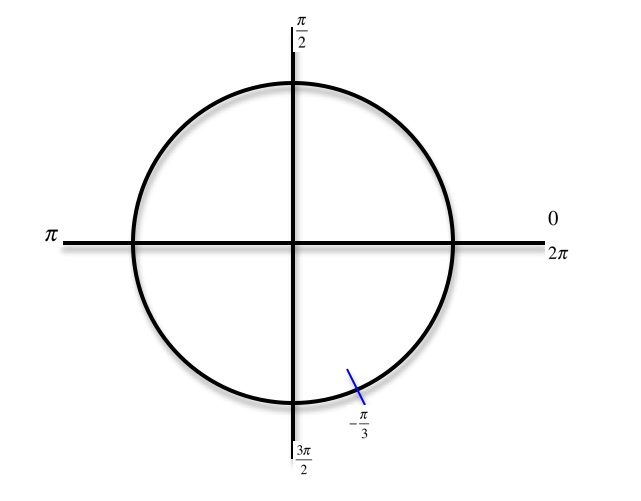• Recall that cosine is the x value of the point you just plotted. Now find the angle that has the same cosine value of − [(π)/3] between 0 and π because 0 ≤ arccos ≤π
• Draw a line across the x - axis to locate a corresponding point that is between 0 and π
•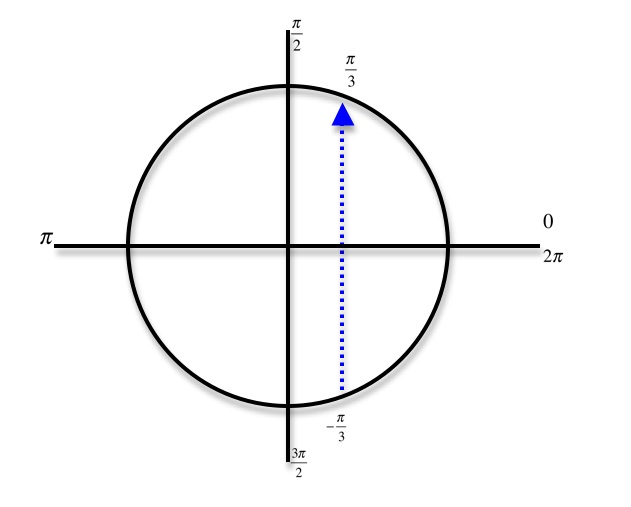arccos[ cos( − [(π)/3]) ] = [(π)/3]
Find arcsin[ sin( − [(4π)/3]) ]
• Plot the point - [(4π)/3] on the unit circle. Note: − [(4π)/3] = − 240°
•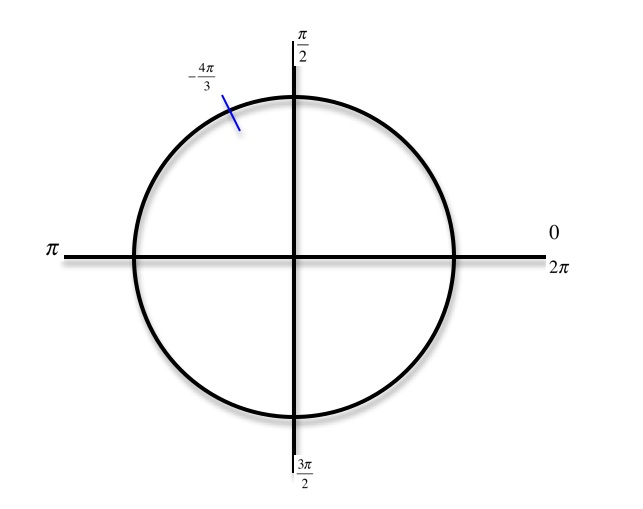• Recall that sine is the y value of the point you just plotted. Now find the angle that has the same sine value of − [(4π)/3] between − [(π)/2] and [(π)/2] because − [(π)/2] ≤ arcsin ≤ [(π)/2]
• Draw a line across the y - axis to locate a corresponding point that is between − [(π)/2] and [(π)/2]
•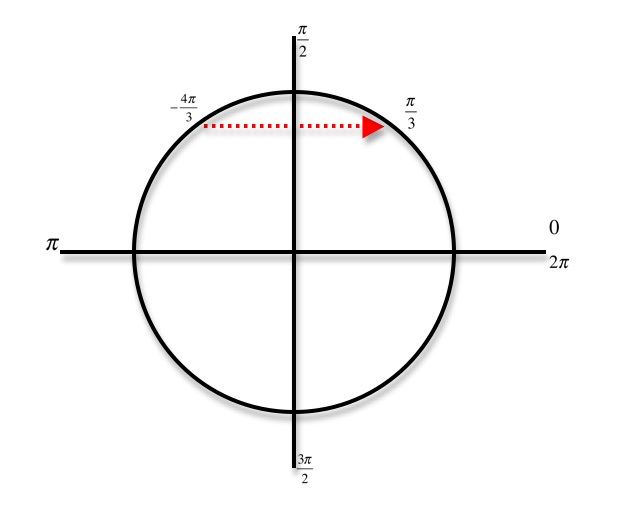arcsin[ sin( − [(4π)/3]) ] = − [(π)/3]
Find arctan[ tan([(5π)/4]) ]
• Plot the point [(5π)/4] on the unit circle. Note: [(5π)/4] = 225°
•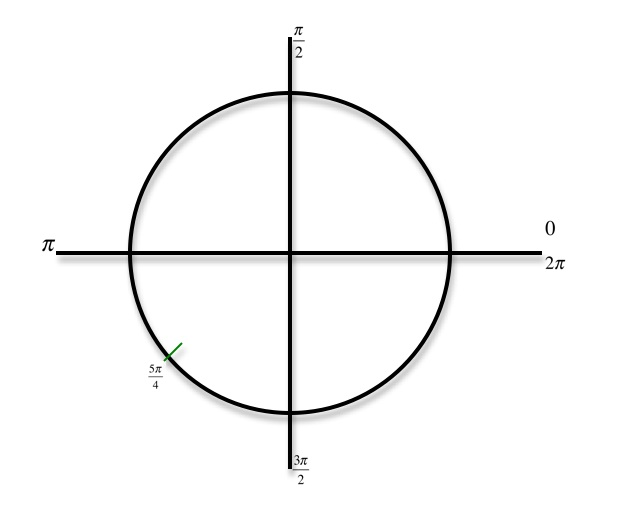• Recall that sine is the y value of the point you just plotted. Now find the angle that has the same tangent value of [(5π)/4] between − [(π)/2] and [(π)/2] because − [(π)/2] ≤ arctan ≤ [(π)/2]
• Draw a line diagonally across to locate a corresponding point that is between − [(π)/2] and [(π)/2]
•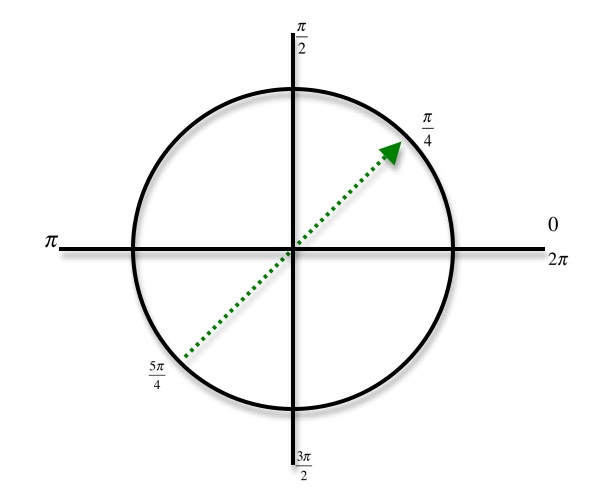arctan[ tan([(5π)/4]) ] = [(π)/4]
Find arccos[ cos([(5π)/4]) ]
• Plot the point [(5π)/4] on the unit circle. Note: [(5π)/4] = 225°
•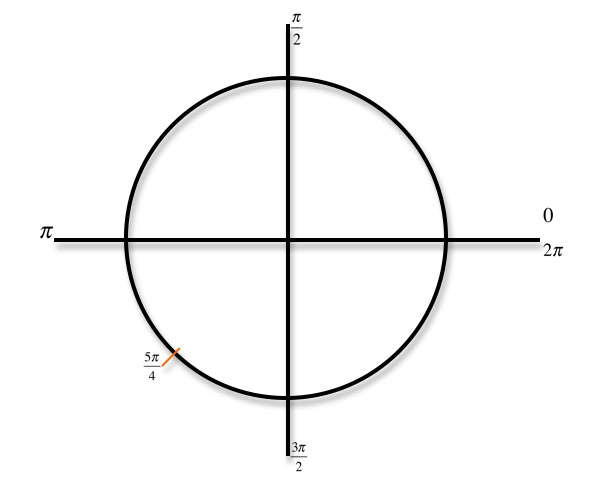• Recall that cosine is the x value of the point you just plotted. Now find the angle that has the same cosine value of [5p/4] between 0 and π because 0 ≤ arccos ≤π
• Draw a line across the x - axis to locate a corresponding point that is between 0 and π
•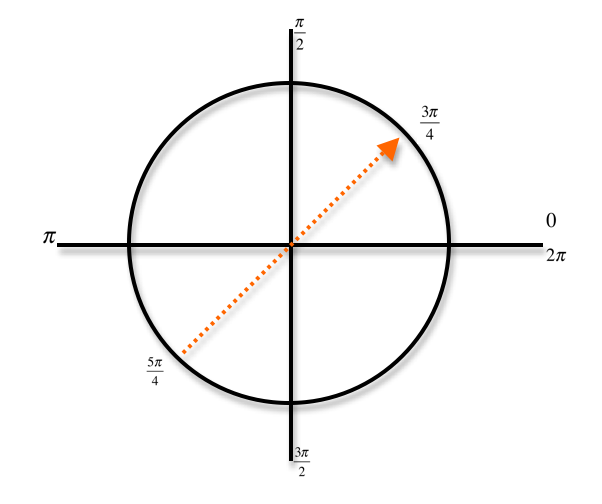arccos[ cos([(5π)/4]) ] = [(3π)/4]
Find arctan[ tan( − [(5π)/6]) ]
• Plot the point − [(5π)/6] on the unit circle. Note: − [(5π)/6] = − 150°
•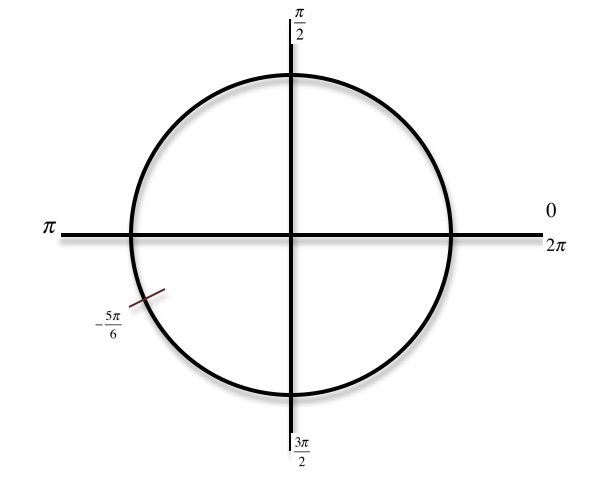• Recall that tangent is [x/y]. Now find the angle that has the same tangent value of − [(5π)/6] between − [(π)/2] and [(π)/2] because − [(π)/2] ≤ arctan ≤ [(π)/2]
• Draw a line diagonally across to locate a corresponding point that is between − [(π)/2] and [(π)/2]
•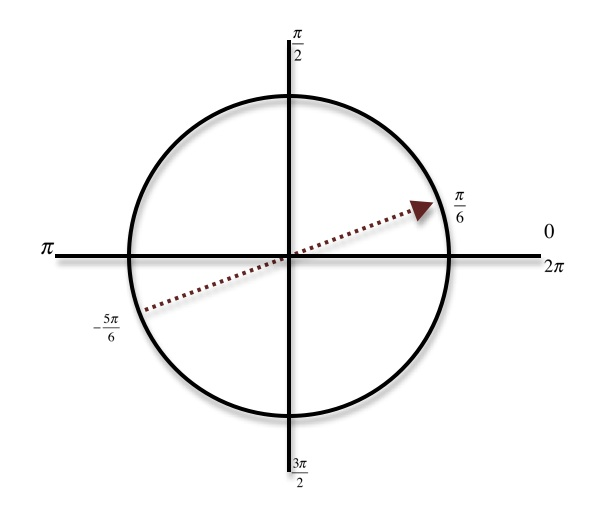arctan[ tan( − [(5π)/6]) ] = [(π)/6]
Find arcsin[ sin( − [(3π)/4]) ]
• Plot the point − [(3π)/4] on the unit circle. Note: − [(3π)/4] = − 135°
•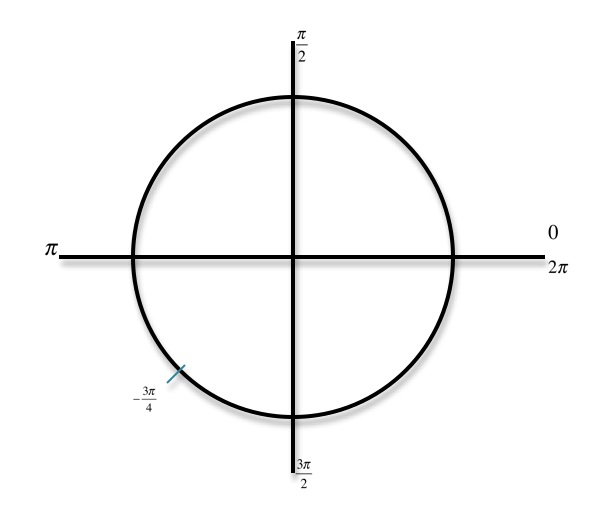• Recall that sine is the y value of the point you just plotted. Now find the angle that has the same sine value of − [(3π)/4] between − [(π)/2] and [(π)/2] because − [(π)/2] ≤ arcsin ≤ [(π)/2]
• Draw a line across the y - axis to locate a corresponding point that is between − [(π)/2] and [(π)/2]
•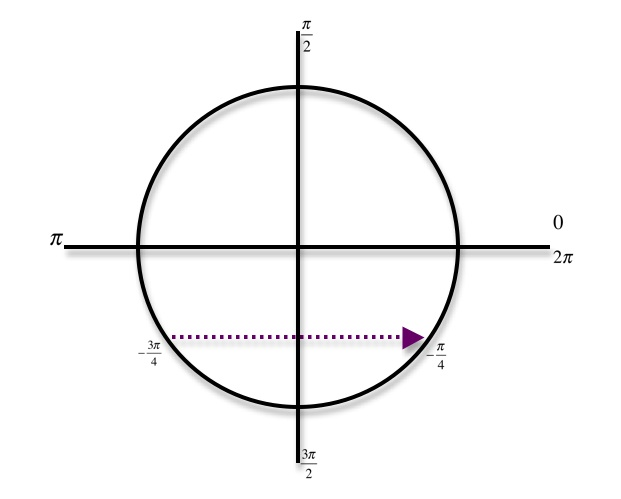arcsin[ sin( − [(3π)/4]) ] = − [(π)/4]
Find arccos[ cos([(7π)/6]) ]
• Plot the point [(7π)/6] on the unit circle. Note: [(7π)/6] = 210°
•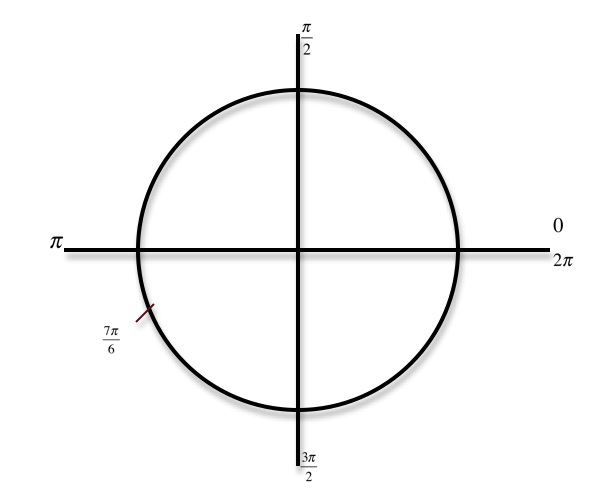• Recall that cosine is the x value of the point you just plotted. Now find the angle that has the same cosine value of [(7π)/6] between 0 and π because 0 ≤ arccos ≤π
• Draw a line across the x - axis to locate a corresponding point that is between 0 and π
•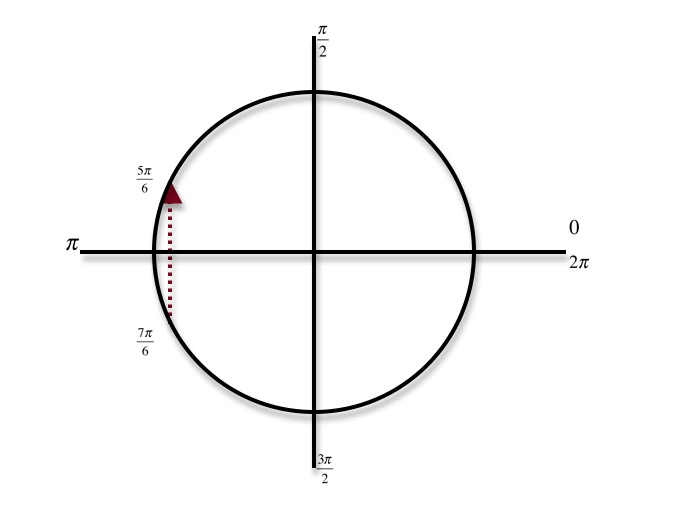arccos[ cos([(7π)/6]) ] = [(5π)/6]
Find arcsin[ sin([(4π)/3]) ]
• Plot the point [(4π)/3] on the unit circle. Note: [(4π)/3] = 240°
•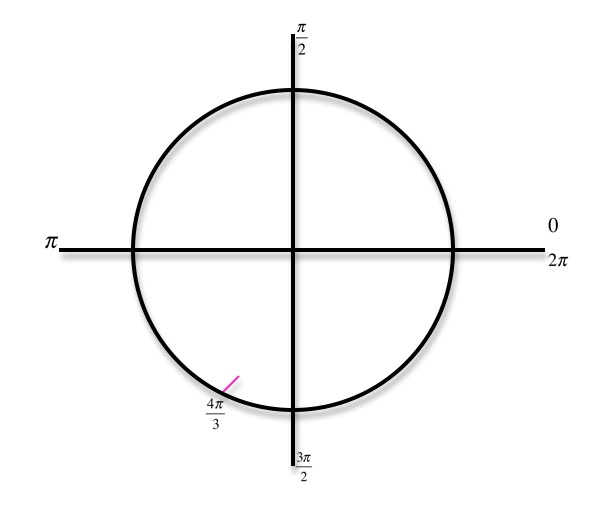• Recall that sine is the y value of the point you just plotted. Now find the angle that has the same sine value of [(4π)/3] between − [(π)/2] and [(π)/2] because − [(π)/2] ≤ arcsin ≤ [(π)/2]
• Draw a line across the y - axis to locate a corresponding point that is between − [(π)/2] and [(π)/2]
•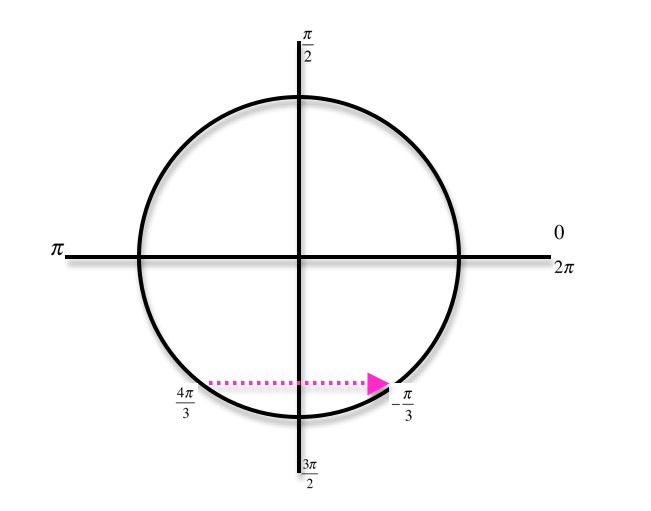arcsin[ sin([(4π)/3]) ] = − [(π)/3]
Find arctan[ tan([(3π)/4]) ]
• Plot the point [(3π)/4] on the unit circle. Note: [3p/4] = 135°
•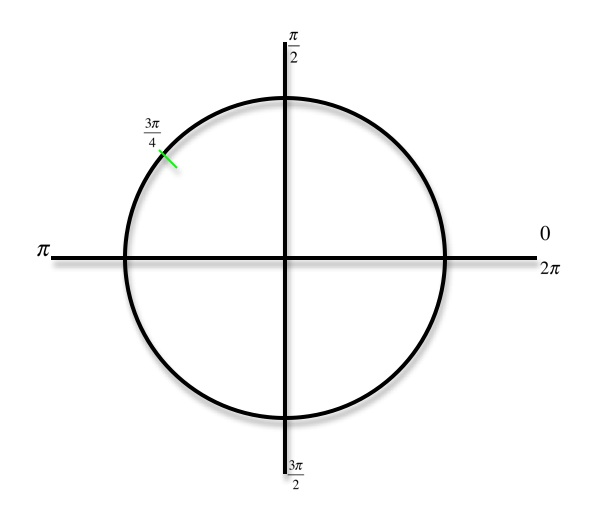• Recall that tangent is [x/y]. Now find the angle that has the same tangent value of [(3π)/4] between − [(π)/2] and [(π)/2] because − [(π)/2] ≤ arctan ≤ [(π)/2]
• Draw a line diagonally across to locate a corresponding point that is between − [(π)/2] and [(π)/2]
•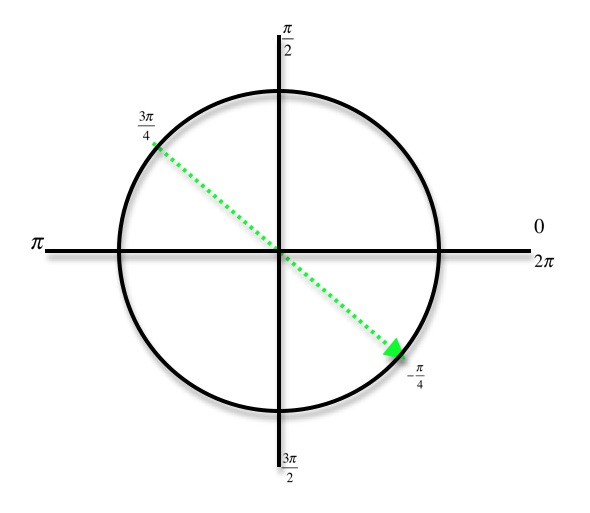arctan[ tan([(3π)/4]) ] = − [(π)/6]
Find arccos[ cos( − [(π)/3]) ]
• Plot the point − [(π)/3] on the unit circle. Note: − [(π)/3] = − 60°
•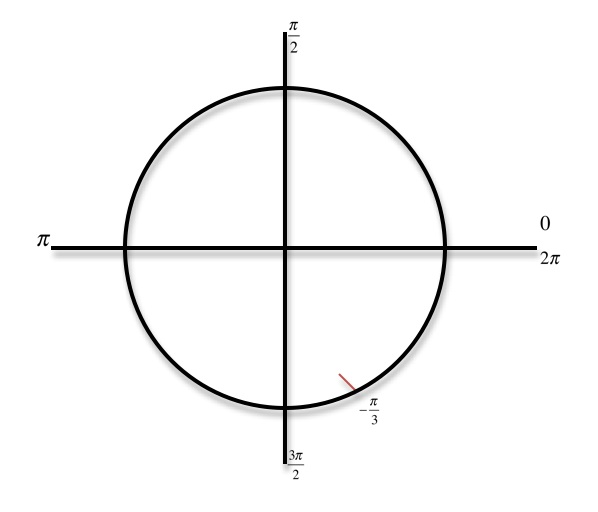• Recall that cosine is the x value of the point you just plotted. Now find the angle that has the same cosine value of − [(π)/3] between 0 and π because 0 ≤ arccos ≤π
• Draw a line across the x - axis to locate a corresponding point that is between 0 and π
•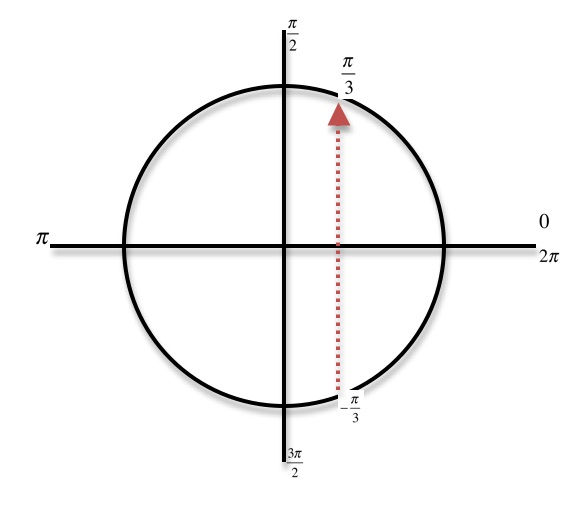arccos[ cos( − [(π)/3]) ] = [(π)/3]

*These practice questions are only helpful when you work on them offline on a piece of paper and then use the solution steps function to check your answer.

### Computations of Inverse Trigonometric Functions

Lecture Slides are screen-captured images of important points in the lecture. Students can download and print out these lecture slide images to do practice problems as well as take notes while watching the lecture.

• Intro 0:00
• Inverse Trigonometric Function Domains and Ranges 0:31
• Arcsine
• Arccosine
• Arctangent
• Example 1: Arcsines of Common Values 2:44
• Example 2: Odd, Even, or Neither 5:57
• Example 3: Arccosines of Common Values 12:24
• Extra Example 1: Arctangents of Common Values
• Extra Example 2: Arcsin/Arccos/Arctan Values

### Transcription: Computations of Inverse Trigonometric Functions

Ok we are trying some more examples of computing values of the inverse trigonometric functions.0000

Here we have to find the arctangent of the following common values.0006

I remember that when arctangent I am always looking for values between –pi/2 and pi/2 because arctangent is always between pi /2 and –pi/ 2.0029

I wrote less than or equal to there, but it should really be less than because arctangent never actually hits – pi/2 or pi/2.0050

That is because tangent itself cannot be evaluated on pi/2 or –pi/2 because you are basically trying to divide by 0 there.0059

I’m going to make a list here of x and arctan z 1 and root 3.0071

It is really useful if you can remember some common values of the tangent function.0098

For example tan (pi/0) is 0.0105

Tan (pi/6) is sine of pi/6 divided by cosine of pi/6, which works out to root 3/30113

Tan (pi/4) is sine of 4 divided by cosine.0126

Well in both are 2/2 so it is one.0132

Tan (pi/3) is sin/cosin of pi/ 3, that is root 3.0136

That tells me what the values of tangent are at these common values, pi/6, pi/4, and pi/3.0143

There is another way to remember the values of the tangent functions, which is to draw this line at x=1.0155

The tangent function is actually where these lines hit that line. That is 1, root 3/3, and root 3.0164

So if you remember your common values of tangent, it is easy to figure out the values of arctangent.0178

Let me start from the bottom because that is the easiest.0186

Root 3 here, what angle has tangent root 3?0190

Well, we just figured that out, that is pi/3.0194

Also, we get that from here.0199

Pi/3 is the angle that has tangent root 3.0201

What angle has tangent 1?0206

Well, there it is right there, it is pi/4.0210

What angle has root 3/3? It is pi/6.0214

From getting these common values over here.0220

What angles has tangent 0? Well, that is 0.0223

The negative ones are little trickier but if you just remember that the values in the fourth quadrant down here.0227

They are just the negatives of the same values we had up in the first quadrant.0235

That is –root 3, -1, and –root 3/3.0242

For –root 3, what is an angle that has that as its tangent? it is –pi/3.0252

For -1, what is an angle that has that as its tangent? It is –pi/4.0259

Finally for –root 3/3, what is an angle that has that as its tangent? It is –pi/6.0264

There are really two things that are keys to being able to find our tangents.0274

The first is knowing your tangents of the common values.0278

Again, if you can not remember those, just remember that tangent is sine over cosine.0282

If you remember the sine and cosine of the common values, it all comes back to those triangles.0295

Then you can figure out the tangents.0299

It is good to remember the common values that you do get from tangent namely root 3, 1, and root 3/3.0302

It is good to remember those numbers. But if you can not remember them, you can work them out from sine and cosine.0311

Once you figure those out, then you can figure out the common values of tangent of the negative angles.0316

You have to remember that arctangent always takes values between –pi/2 and pi/2.0324

Looking for angles between –pi/2 and pi/2 that have the tangents that we have been given.0334

When we are given these tangents, we just remember values between –pi/2 and pi/2 that have those particular tangents.0341

On our last example here, we are given various angles.0000

We have to find the arcsine of their sine or the same for cosine and tangent.0005

It is very important that you would be able to graph this on the unit circle.0012

That is really the way you start the problem like this.0015

There is my unit circle and that is 0, pi/2, pi, 3 pi/2, and 2 pi.0021

Now, -7 pi/6. The negative means you are going the downward direction from 0.0040

If you go a little bit past pi and -7 pi/6 puts you right there, -7 pi/6.0046

So, arcsine of sine of -7 pi/60056

The sine of that angle is the y coordinate and it is positive there.0068

So, it is arcsine and that is a 30, 60, 90 triangle.0076

I know the common values there are ½. It is positive because the y coordinate is positive.0083

It is arcsine of 1 ½ but remember that arcsine is always between – pi/2 and pi/2.0088

I want an angle between –pi/2 and pi/2.0105

Whose sign is ½. Well, sign is the y coordinate.0108

There is an angle who is y coordinate is ½ and that angle is pi/6.0117

My x are, there is pi/6.0126

It is an angle between –pi/2 and pi/2 who is sign is ½.0132

Let us try the next one, arcosine of 4 pi/3.0138

Well, I find 4 pi/3, that is between pi and 2 pi.0148

In fact, that is down here.0153

Its cosine is x coordinate, its cosine is x coordinate and there again we have a 30, 60, 90 triangle.0158

Its cosine of 4 pi/3 is ½.0174

But since we are on the left side of the page, it is negative because the x coordinate is negative there.0183

Now I’m trying to find the arcosine of – ½.0189

Remember in arcosine, you will always look for an angle between 0 and pi.0196

I’m looking for an angle between 0 and pi whose cosine is – ½.0200

There it is right there.0209

That is 2 pi/3.0212

It is between 0 and pi, and its cosine is – ½.0215

So, our answer there is 2 pi/3.0219

Finally, we have the arctangent of the tangent of -5 pi/4.0229

Let us figure out where that angle is on the unit circle.0239

It is negative so we are going around in the downward direction from 0.0242

5 pi/4 is just past pi.0246

We go down on the downward direction and we end up over here at 5 pi/4.0250

So, -5 pi/4. I’m sorry that is -5 pi/4. Let me go back carefully.0260

Its tangent is sine/cosine.0270

That is a 45, 45, 90 triangle.0276

Its tangent is sine/cosine.0285

Sine is root 2/2 because it is positive.0288

Cosine is –root 2/2 that simplifies down to -1.0293

We want the arctangent of -1.0299

Now, arctangent always takes values between -pi/2 and pi/2 arctangent.0302

We are looking for an angle between –pi/2 and pi/2 whose tangent is -1.0317

So, I’m looking for an angle whose tangent is -1.0327

There it is at that 45, 45, 90 triangle.0339

There is my angle whose tangent is -1.0344

And that angle is –pi/4.0348

To recap, what really made it possible to this problem were finding sine, cosine, and tangent to start with.0361

The first thing you need to is your common values of sine, cosine, and tangent.0369

You need to be able to graph these things on the unit circle.0374

You need to identify the 30, 60, 90 triangles and the 45, 45, 90 triangles and know the common values.0378

Remember the common values for the 30, 60, 90 triangles are always ½ and root 3/2.0388

The common values for a 45, 45, 90 triangles are root 2/2 and root 2/2.0398

You need to know those common values and identify which triangle you are working with.0414

And figure out what quadrant you are in to figure out whether the sine and cosine are positive or negative.0423

The way you remember that is by all students take calculus.0430

In the first quadrant, they are all positive.0434

In the second quadrant, sine is positive only.0437

In the third quadrant, tangent is positive.0440

And in the fourth quadrant, cosine is positive.0443

You figure out which quadrant all these things are positive or negative and then you can find your sine and cosine.0448

And then what we have to do is find arcsines, arcosines, and arctangents.0456

The key to that was remembering the common values but also remembering these ranges.0462

Arcsine, it has to be between –pi/2 and pi/2.0470

Arcosine, between 0 and pi.0474

And arctangent, between –pi/2 and pi/2.0477

What we are doing in this step for all three problems was we had the sine or the cosine of the tangent.0482

We were trying to find an angle in the appropriate range that had the correct sine, cosine, or tangent.0490

That is where our arcosine, arsine, and arctangent are.0498

We are looking for an angle whose sign, cosine, and arctangent is the value that you are given.0501

We are looking for an angle inside this range.0510

In each case, we have a value and we found angles inside that range that have the right sine, cosine, or tangent.0514

That is what the answers are.0521

That is the end of our lecture on computations of inverse trigonometric functions.0524

These are the trigonometry lectures for www.educator.com.0529

Hi these are the trigonometry lectures for educator.com and today we're talking about computations of inverse trigonometric functions.0000

In the previous lecture, we learned the definitions and we practiced a little bit with arcsin, arccos, and arctan, you might want to review those a little bit before you go through this lecture.0009

I'm assuming now that you know a little bit about the definitions of arcsin, arccos, and arctan, and we'll practice using them and working them out for some common values today.0020

The key thing to remember here is where these functions are defined and what kinds of values you're going to get from them.0032

Arcsin, remember you start out with the number between -1 and 1, and you always get an answer between -π/2 and π/2.0040

It's very helpful if you remember the unit circle there.0052

Arcsin always gives you an angle in the fourth and the first quadrant between -π/2, 0, and π/2.0055

You're looking for angles in that range that have a particular sine.0068

Arccos, also between -1 and 1, produces an answer between 0, and π.0075

Again, it's helpful to draw the unit circle and keep that in mind.0081

There's 0, π/2, and π.0088

You're trying to find angles between 0 and π that have a given cosine.0095

Arctan, you can find the arctan of any number.0101

Again, you're trying to find an angle between -π/2 and 0, and π/2 that has a given tangent.0110

I say exclusive here because you would never actually give an answer of -π/2 or π/2 for arctan because arctan never actually hits those values.0129

If you think of that coming from the other direction, we can't talk about the tangent of π/2 or -π/2, because those involve divisions by 0.0143

When we're talking about arctan, we'll never get -π/2 or π/2 as an answer.0153

Let's practice finding some common values.0161

Here's some common values that we should be able to figure out arc sines of.0166

Let me start by drawing my unit circle.0172

There's -π/2 and 0, and π/2, remember, our answers always going to be in that range.0183

Let me just graph those common values and see what angles they correspond to.0191

I'll make a little chart here.0197

x and arcsin(x), so we got -1, negative root 3 over 2, negative root 2 over 2, -1/2, 0, 1/2, root 2 over 2, root 3 over 2, and 1.0198

Remember, sin(x), sine is the y-coordinate.0222

I'm looking for an angle that has y-coordinate of -1 to start with, so I want to find an angle that has y-coordinate down there at -1, and that's clearly -π/2.0227

Negative root 3 over 2, that's an angle down there, so the angle that has sine of negative root 3 over 2, must be -π/3.0240

Negative root 2 over 2, that's the one right there, so that's a -π/4.0258

-1/2, the y-coordinate -1/2, is right there, that's -π/3.0267

Arcsin(0), what angle has sin(0)?0277

Well, it's 0.0280

What angle has sin(1/2)?0283

Well, what angle has vertical y-coordinate 1/2?0285

That's π/3.0288

Root 2 over 2, that's our 45-degree angle, also known as π/4.0294

Root 3 over 3, that's our 60-degree angle, also known as π/3.0303

Finally, we know that the sin(π/2) is 1, so the arcsin(1)=π/2.0313

In each case, it's a matter of looking at the value and thinking, "Okay, that's my y-coordinate, where am I on the unit circle?"0321

"What angle between -π/2 and π/2 has sine equal to that value?"0332

Of course, if you know your values of sine very well, then it's not too hard to figure out the arcsin function.0339

You really don't need to memorize this.0345

You just need to know your common values for sin(x) very well, and to know when they're positive or negative.0348

Then you can figure out the values for arcsin(x).0354

In our second problem here, we're asked to find which of the arcsin, acrccos, and arctan functions are odd, even or neither.0360

It's really, the key to thinking about this one is probably to think about the graphs and not so much to think about the original definitions of odd or even.0369

Let me write down the important properties to remember here.0377

An odd function has rotational symmetry around the origin.0382

The way I remember that is that 3 is an odd number and x3, y=x3 has rotational symmetry around the origin.0406

Even functions have mirror symmetry across the y-axis and the way I remember that is that 2 is an even number, and the graph of y=x2 has mirror symmetry across the y-axis.0423

That's how I remember the pictures for odd or even functions.0458

Now, let me draw the graphs of arcsin and arccos, and arctan, and we'll just test them out.0464

Arcsin, remember you take a piece of the sine graph, there's sin(x) or sin(θ).0473

Arcsin, I'll draw this one in blue.0482

It's the reflection of that graph in the y=x line, that's arcsin(x) in blue.0485

Now, if you check that out, that has rotational symmetry around the origin.0497

So, arcsin(x) is odd.0507

Let's take a look at cosine and arccos.0517

Cosine, remember, you got to snip off a piece of cosine graph that will make arccos into a function.0522

There's cos(θ), and now in blue, I'll graph arccos.0530

There's arccos(x) in blue.0546

Now, that graph is neither mirror symmetric across the y-axis nor is it rotationally symmetric around the origin.0552

So, it's not odd or even, which is a little bit surprising, because even though if you remember cos(θ) is even.0565

It turns out that arccos(x) is not odd or even, even though cos(θ) was an even function.0590

Finally, arctan, let me start out by drawing the tangent graph, or at least the piece of it that we're going to snip off.0597

It kind of looks like the graph of y=x3, but it's not the same as the graph of y=x3.0609

One big difference is that tan(x) has asymptotes at π/2 and -π/2, and of course y=x3 has no asymptotes at all.0617

What I just graphed here is tan(θ) and then in blue I'll graph arctan(θ).0627

I'm flipping it across the line y=x.0638

It also has asymptotes neither horizontal asymptotes.0642

That blue graph is arctan(x), and if you look at that, that is rotationally symmetric around the origin.0648

So that's also an odd function.0666

This problem is really kind of testing whether you know the graphs of arcsin, arccos, and arctan look like.0675

If you don't remember those, then you go back to sine, cosine and tangent, and you snip off the important pieces of those graphs, and you flip them around y=x to get the graphs of arcsin, arccos, and arctan.0685

Those are the graphs that I have in blue here, arcsin, arccos, and arctan.0700

The other thing that this problem is really testing is whether you remember the graphical characterizations of odd and even functions.0705

If you know that odd functions have rotational symmetry around the origin, even functions have mirror symmetry across the y-axis, it's easy to check these graphs to just look at them and see whether they have the right kind of symmetry.0717

Of course, what you find out is that arcsin(x) has rotational symmetry, arccos(x) doesn't have either one, arctan(x) also has rotational symmetry.0732

For our third example here, we're trying to find arccos of the following list of common values.0746

Once you start with a unit circle, remember that with arccos, you're looking for values between 0 and π.0766

Arccos is always between 0 and π.0779

We're looking for angles between 0 and π that have cosines equal to this list of values.0786

I'll make a little chart here.0796

-1, negative root 3 over 2, negative root 2 over 2, -1/2, 0, 1/2, root 2 over 2, root 3 over 2 and 1.0805

Remember, cosine is the x-value.0823

I'm going to draw each one of these values as an x-value, as an x-coordinate, and then I'll see what angle has that particular cosine.0826

So, -1, the x-coordinate of -1 is over here, clearly that's π, that's the angle π.0834

Negative root 3 over 2, I'll draw that as the x-coordinate, and that angle is 5π/6.0845

Negative root 2 over 2, I'll draw that as the x-coordinate, I know that's a 45-degree angle, so that's 3π/4, that's the arccos of negative root 2 over 20863

-1/2, if we draw that as the x-coordinate, that's a 30-60-90 triangle, that's 2π/3.0879

What angle has cos(0)?0894

That means what angle has x-coordinate 0, that's π/2.0896

What angle has cos(1/2)?0903

Again, a 30-60-90 triangle, that must be π/3.0909

Root 2 over 2, that's a 45-degree angle, so that's π/4.0917

Root 3 over 2, that's a 30-60-90 angle again, that's π/6.0927

Finally, what angle has x-coordinate 1?0939

That's just 0.0943

The trick here is remembering your common values of cosine on the unit circle.0947

I know all the common values of cosine on the unit circle very well because I remember my 30-60-90 triangles, and I remember my 45-45-90 triangles.0952

I know which ones are positive and which ones are negative.0965

Finally, I remember that arccos is always between 0 and π.0968

So, I'm looking for angles between 0 and π that have these cosines.0973

These are the angles that have the right cosines and are in the right range.0981

We'll try some more examples of these later.0985

OR

### Start Learning Now

Our free lessons will get you started (Adobe Flash® required).